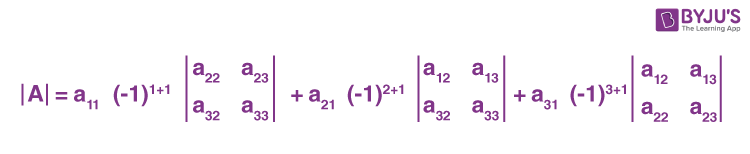# Determinant of a 3 x 3 matrix

In matrices, determinants are the special numbers calculated from the square matrix. The determinant of a 3 x 3 matrix is calculated for a matrix having 3 rows and 3 columns. The symbol used to represent the determinant is represented by vertical lines on either side, such as | |. The most popular application is to find the area of a triangle using a determinant, where the three vertices of the triangle are considered as the coordinates in an XY plane.

Let A be the matrix, and then the determinant of a matrix A is denoted by |A|. To find the determinant of matrices, the matrix should be a square matrix, such as a determinant of 2×2 matrix, determinant of 3×3 matrix, or n x n matrix. It means the matrix should have an equal number of rows and columns. Finding determinants of a matrix is helpful in solving the inverse of a matrix, a system of linear equations, and so on. In this article, let us discuss how to solve the determinant of a 3×3 matrix with its formula and examples.

## Determinant of a 3 x 3 Matrix Formula

We can find the determinant of a matrix in various ways. First, we have to break the given matrix into 2 x 2 determinants so that it will be easy to find the determinant for a 3 by 3 matrix.

We can find the determinant of a matrix in various ways. First, we have to break the given matrix into 2 x 2 determinants so that it will be easy to find the determinant for a 3 by 3 matrix.

Let’s suppose you are given a square matrix A where

$$\begin{array}{l}A=\begin{bmatrix}a_{11} & a_{12} & a_{13} \\a_{21} & a_{22} & a_{23} \\a_{31} & a_{32} & a_{33} \\\end{bmatrix}\end{array}$$

Let’s calculate the determinant of matrix A, i.e., |A|.

$$\begin{array}{l}|A|=\begin{vmatrix}a_{11} & a_{12} & a_{13} \\a_{21} & a_{22} & a_{23} \\a_{31} & a_{32} & a_{33} \\\end{vmatrix}\end{array}$$

By expanding along the first row elements, we get;

$$\begin{array}{l}|A|=\begin{vmatrix}a_{11} & a_{12} & a_{13} \\a_{21} & a_{22} & a_{23} \\a_{31} & a_{32} & a_{33} \\\end{vmatrix}=a_{11}(-1)^{1+1}\begin{vmatrix}a_{22} & a_{23} \\a_{32} & a_{33} \\\end{vmatrix}+a_{12}(-1)^{1+2}\begin{vmatrix}a_{21} & a_{23} \\a_{31} & a_{33} \\\end{vmatrix}+a_{13}(-1)^{1+3}\begin{vmatrix}a_{21} & a_{22} \\a_{31} & a_{32} \\\end{vmatrix}\end{array}$$

This can be written as:

$$\begin{array}{l}|A|=\begin{vmatrix}a_{11} & a_{12} & a_{13} \\a_{21} & a_{22} & a_{23} \\a_{31} & a_{32} & a_{33} \\\end{vmatrix}=a_{11}\begin{vmatrix}a_{22} & a_{23} \\a_{32} & a_{33} \\\end{vmatrix}-a_{12}\begin{vmatrix}a_{21} & a_{23} \\a_{31} & a_{33} \\\end{vmatrix}+a_{13}\begin{vmatrix}a_{21} & a_{22} \\a_{31} & a_{32} \\\end{vmatrix}\end{array}$$

Therefore,

 |A| = a11 (a22 a33 – a23 a32) – a12 (a21 a33 – a23 a31) + a13 (a21 a32 – a22 a31)

Writing the determinant of a 3×3 matrix – Simple points

To find the determinant of a 3×3 matrix, first we need to find the minor matrices of any row or column elements. Suppose, we want to find the determinant of the matrix A by expanding the first column elements, then write the determinants of 2×2 matrices obtained by eliminating the corresponding elements’s row & column.

For example,

$$\begin{array}{l}|A|=\begin{vmatrix}a_{11} & a_{12} & a_{13} \\a_{21} & a_{22} & a_{23} \\a_{31} & a_{32} & a_{33} \\\end{vmatrix}\end{array}$$

For element a11, we can find the 2×2 scalar matrix as follows.For the element a21, the scalar matrix is obtained as:Similarly, for the element a31, the 2×2 scalar matrix is obtained as follows:Thus, the determinant of matrix A is written as:## Solved Examples

Let’s understand the calculation of determinant of 3×3 matrices with the help of solved examples below:

Example 1: Calculate the determinant of the 3×3 matrix given below:

$$\begin{array}{l}A=\begin{bmatrix}1 & -1 & 0 \\2 & 3 & 4 \\0 & 1 & 2 \\\end{bmatrix}\end{array}$$

Solution:

Let’s compare the given matrix with the general matrix form of the 3×3 matrix.

That means,

$$\begin{array}{l}A=\begin{bmatrix}a_{11} & a_{12} & a_{13} \\a_{21} & a_{22} & a_{23} \\a_{31} & a_{32} & a_{33} \\\end{bmatrix}=\begin{bmatrix}1 & -1 & 0 \\2 & 3 & 4 \\0 & 1 & 2 \\\end{bmatrix}\end{array}$$

Here,

a11 = 1, a12 = -1, a13 = 0

a21 = 2, a22 = 3, a23 = 4

a31 = 0, a32 = 1, a33 = 2

Thus, by applying the determinant of a 3×3 matrix formula, we have

$$\begin{array}{l}|A|=1.\begin{vmatrix}3 & 4 \\1 & 2 \\\end{vmatrix}-(-1).\begin{vmatrix}2 & 4 \\0 & 2 \\\end{vmatrix}+0.\begin{vmatrix}2 & 3 \\0 & 1 \\\end{vmatrix}\end{array}$$

= 1 [3(2) – 1(4)] + 1[2(2) – 4(0)] + 0

= (6 – 4) + (4 – 0)

= 2 + 4

= 6

Example 2:

Find the determinant of the following 3×3 matrix.

$$\begin{array}{l}P=\begin{bmatrix}0 & 1 & 1 \\1 & 1 & 0 \\1 & 0 & 1 \\\end{bmatrix}\end{array}$$

Solution:

Let’s compare the given matrix with the general matrix form of the 3×3 matrix.

That means,

$$\begin{array}{l}\begin{bmatrix}a_{11} & a_{12} & a_{13} \\a_{21} & a_{22} & a_{23} \\a_{31} & a_{32} & a_{33} \\\end{bmatrix}=\begin{bmatrix}0 & 1 & 1 \\1 & 1 & 0 \\1 & 0 & 1 \\\end{bmatrix}\end{array}$$

Here,

a11 = 0, a12 = 1, a13 = 1

a21 = 1, a22 = 1, a23 = 0

a31 = 1, a32 = 0, a33 = 1

Now, by applying the determinant of a 3×3 matrix formula, we get;

$$\begin{array}{l}|A|=0.\begin{vmatrix}1 & 0 \\0 & 1 \\\end{vmatrix}-1.\begin{vmatrix}1 & 0 \\1 & 1 \\\end{vmatrix}+1.\begin{vmatrix}1 & 1 \\1 & 0 \\\end{vmatrix}\end{array}$$

= 0 -1 [1(1) – 0(1)] + 1[1(0) – 1(1)]

= -1(1) + (-1)

= -1 – 1

= -2

Found this topic interesting, explore more on other mathematical topics on BYJU’S- The Learning App.

 Learn more on Matrices Determinant Formula Inverse Matrix Formulas Determinants and Matrices Determinants for Class 12